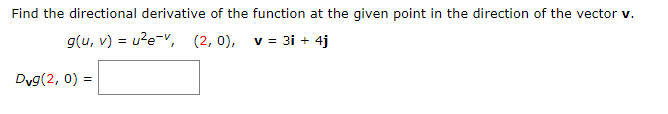# Find the directional derivative of the function at the given point in the direction of the vector v. g(u, v) = u?e=v, (2, 0), v = 31 + 4j Dyg(2, 0) =

Questionhelp_outlineImage TranscriptioncloseFind the directional derivative of the function at the given point in the direction of the vector v. g(u, v) = u?e=v, (2, 0), v = 31 + 4j Dyg(2, 0) = fullscreen

### Want to see this answer and more?

Experts are waiting 24/7 to provide step-by-step solutions in as fast as 30 minutes!*

*Response times may vary by subject and question complexity. Median response time is 34 minutes for paid subscribers and may be longer for promotional offers.
Tagged in
Math